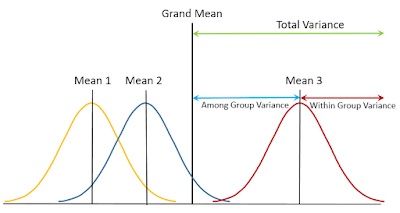### Using Analysis of Variance (ANOVA) in ecological research

Analysis of Variance (ANOVA) is one of the most commonly applied statistical analysis in ecological research.  Many scientists in ecology area use ANOVA to test whether averages of more than two groups are same or not.  It is an extension of t-test which tests whether averages of two groups are same or not.  For example, let us assume that we are testing averages of three different group.  If averages of all three groups are not so different, the variance among groups (variance for three average values) would be very small. However, you also consider variances within group (variance for each data from their group average).Fig. 1 Concept for analysis of variance (ANOVA) showing total variance is sum of variance for among groups and variance for within group.   ©S Park

If the among group variance is large enough compared to within group variance, we may say that averages of these three groups are significantly different.  To test the null hypothesis ("Three group averages are same"), we simply need to calculate the ratio of among group variance to within group variance, F value.  As variance ratio follow F-distribution, we can determine if a F value is large enough to reject the null hypothesis at 95% confidence level.Fig. 2 Examples for a case with averages of three groups are not considerably different (above) and a case with averages are considerably different (below). ©S Park

Let's build an ANOVA table.Fig. 3 ANOVA table structure. ©S Park

First, you have to calculate sum of squares (SS), which show variance for among groups and within groups. As we already know, total variance (sum of squares) is sum of SS among groups and SS within groups. If you divide each SS by their own degree of freedom (df), you will get mean squares (MS) among groups and between groups.  F is just the ratio of MS among groups to MS within groups.

Now, let's look a real ANOVA table from Journal of Ecology and Environment.Fig. 4 An ANOVA table from an ecological research paper (Cho et al.: Distribution and synchronized massive flowering of Sasa borealis in the forests of Korean National Parks. Journal of Ecology and Environment 2018, 42:37)
ANOVA table above comes from an ecological research paper regarding synchronized massive flowering of dwarf bamboo (Sasa borealis) (Cho et al. 2018). It combined three independent ANOVA results (Culm density, Culm height, and Culm diameter) showing two among group variances for each ANOVA (within groups variances were not shown).   All three ANOVA are 2-way since each ANOVA has two among group variances.  The ANOVA table above indicates that Culm density, height, and diameter are significantly different among National Parks while Culm height and diameter are significantly different among flowering types.  You can verify the pattern that higher F values lead lower p values in the ANOVA table above.

It is worth keeping in mind that ANOVA is not just for statistical test on differences among groups.  More important and usually ignored side of ANOVA may be showing variance explained by each variables. ANOVA is literally "ANalysis Of VAriance" showing variances assigned by among groups, that is your variables (different regions, flowering type, etc.) and residuals (individual errors)  Let's look at another ANOVA table.Fig. 4 ANOVA table from an ecological research paper (Kim et al.: Genetic variation and structure of Juniperus chinesis L. (Cupressanceae) in Korea. Journal of Ecology and Environment 2018, 42:14)

This ANOVA table above comes from an ecological research paper regarding genetic variation and structure of chinese juniper (Juniperus chinensis) (Kim et al. 2018). Although this table is called AMOVA (Analyis of molecular variance), the underlying principle is essentially same as ANOVA. The table shows two among group variances for each ANOVA and within group variance (individual), indicating 2-way ANOVA. The most important variable in ANOVA table is sum of squares (SS) which show the contributions of each source of variation.  Therefore, you can calculate percentage of variations due to each source of variation by dividing SS of each source by total SS multiplied by 100.  Most ANOVA table lack the information on how each source of variation contribute to the total variation.  Fortunately, the ANOVA table above shows "Percentage of variation", indicating that variance within groups (individual) explains most (83%) of the total variance at the ISSR loci.  Ecologists should keep in mind that the contributions of the treatment (among group variation) is important quantities to check in addition to statistical significance (F and p values).  Is it all right to interpret a factor as important if p value indicate significance at 95% level although among group variance for the factor explains only 10% of the total variance?

by Sangkyu Park (Ajou University)

References
Kim E-H, Shin J-K, J K-S, Lee C-S, Chung J-M: Genetic variation and structure of Juniperus chinesis L. (Cupressanceae) in Korea. Journal of Ecology and Environment 2018, 42:14

Cho S, Y Kim, Y Choung: Distribution and synchronized massive flowering of Sasa borealis in the forests of Korean National Parks. Journal of Ecology and Environment 2018, 42:37